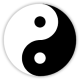# DAY

### Finding the Day Master and Animal

In Chinese Astrology the Day column is the most important column in a person’s chart. The Heavenly Stem of the Day, called the Day master is the person itself. Every other element in the Four Column builds upon this Day Master, showing the different structures and favourable or unfavourable directions in the chart, that goes towards building a more complete horoscope analysis.

The Day Element and Animal can be found easily by working out the below few steps:

1. First look up the code number for the Year in Table A.
2. Then find the code number of the Month from Table B.
3. Now add together the code number of the Year and the Month, plus the day of the birth.
4. If the year is a leap year (the last two digits of the year can be divided by 4, or see the Leap Years Table below) and the birth date is from March onwards, add 1 more number (for the fact that there are only 29 days in February in leap years).
5. If the total of the found number is less than 60 find the Day element and Animal from Table C.
6. If the total is more than 60, subtract 60 then find the Day element and Animal from Table C.
7. If the total is more than 120, subtract 120 and find the Day element and animal from Table C.

Example 1: Day element and Animal for 29 August 1984.

1. Find from Table A the code number for 1984. (30)
2. From Table B find the code number for August. (32)
3. Code number A = 30; code number B = 32; birth day = 29

30 + 32 + 29 = 91.

1. 1984 was a leap year so add 1 more.

91 + 1 = 92

1. The total is more than 60, so subtract 60.

92 – 60 = 32

1. Therefore 29 August 1984 is a Yin Wood Sheep day.

Example 2: Day element and animal for 31 December 1989

1. Find from Table A the code number for 1989. (57)
2. From Table B find the code number for December. (34)
3. Code number A = 30; code number B = 32; birth day = 31

57 + 34 + 31 = 122.

1. 1989 was not a leap year so no need to add 1 more.
2. The total is more than 120, so subtract 120.

122 – 120 = 2

1. Therefore 31 December 1989 is a Yin Wood Ox day.Leap Years 1800 – 2400 1804 1904 2004 2104 2204 2304 1808 1908 2008 2108 2208 2308 1812 1912 2012 2112 2212 2312 1816 1916 2016 2116 2216 2316 1820 1920 2020 2120 2220 2320 1824 1924 2024 2124 2224 2324 1828 1928 2028 2128 2228 2328 1832 1932 2032 2132 2232 2332 1836 1936 2036 2136 2236 2336 1840 1940 2040 2140 2240 2340 1844 1944 2044 2144 2244 2344 1848 1948 2048 2148 2248 2348 1852 1952 2052 2152 2252 2352 1856 1956 2056 2156 2256 2356 1860 1960 2060 2160 2260 2360 1864 1964 2064 2164 2264 2364 1868 1968 2068 2168 2268 2368 1872 1972 2072 2172 2272 2372 1876 1976 2076 2176 2276 2376 1880 1980 2080 2180 2280 2380 1884 1984 2084 2184 2284 2384 1888 1988 2088 2188 2288 2388 1892 1992 2092 2192 2292 2392 1896 1996 2096 2196 2296 2396 2000 2400

## 2 thoughts on “DAY”

1.jet says:

What is the formula for the years to know the day master?

Like

2.mychineseastrology89 says:

Hi Jet

The formula for the Day Pillar is a bit complicated, that’s why I didn’t included above but here it is:

Day Pillar calculation formula:

DP = R of [(*Y × 5) + (*Y / 4) + 9 + *D + BM + I] / 60
Where R = remainder
Y = last two digits of the year (if year is 1900–1999)
Y = last two digits of the year + 100 (if year is 2000–present)
*Y = Y – 1 if birth month is January or February
*D = the day of birth
BM = the number of “big months” passed, starting in March (Note: “Big months” = all months that have 31 days)
I = 30 if the month is an “even” month (using the Gregorian calendar number system)
I = 0 if the month is an “odd” month (using the Gregorian calendar number system)

You will also need a so called Sexagenary Cycle Chart that I’m happy to send it to you via email as I’m not able to paste it here (please write to mychineseastrology89@gmail.com) as . after you found the R remainder from above calculation you’ll need this chart where you can look for your Day Pillar (Day Master and animal) based on this number.

Like